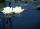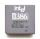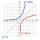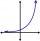After 548 hours decreases the activity of a radioactive substance to 1/9 of the initial value. What is the half-life of the substance?

Result

t1/2 =  172.9 hours

Solution:Leave us a comment of example and its solution (i.e. if it is still somewhat unclear...):Be the first to comment!Next similar examples:

1. Theorem proveWe want to prove the sentence: If the natural number n is divisible by six, then n is divisible by three. From what assumption we started?
2. Logif ?, what is b?
3. The city 3The city has 22,000 residents. How long it is expected to have 25,000 residents if the average annual population growth is 1.4%?
4. Exponential equationIn the set R solve the equation: ?
5. Intercept with axisF(x)=log(x+4)-2, what is the x intercept
6. Water liliesWater lilies are growing on the pond and their number is doubled every day. The whole layer is covered in 12 days. How many days will it cover 8 layers?
7. LogarithmDetermine the number whose decimal logarithm is -3.8.
8. Computer revolutionWhen we started playing with computers, the first processor, which I remember was the Intel 8080 from 1974, with the performance of 0.5 MIPS. Calculate how much percent a year rose CPU performance when Intel 486DX from 1992 has 54 MIPS. What
9. SequenceCalculate what member of the sequence specified by ? has value 86.
10. CoordinateDetermine missing coordinate of the point M [x, 120] of the graph of the function f bv rule: y = 5x
11. Exponential equationSolve exponential equation (in real numbers): 98x-2=9
12. Powers 32 to the power of n divided by 4 to the power of -3 equal 4. What is the vaule of n?
13. Car valueThe car loses value 15% every year. Determine a time (in years) when the price will be halved.
14. Compound interestCalculate time when deposit in the bank with interest 2.5% p.a. doubles.
15. TrinityHow many different triads can be selected from the group 43 students?
16. SequenceBetween numbers 1 and 53 insert n members of the arithmetic sequence that its sum is 702.
17. Insert into GPBetween numbers 5 and 640 insert as many numbers to form geometric progression so sum of the numbers you entered will be 630. How many numbers you must insert?